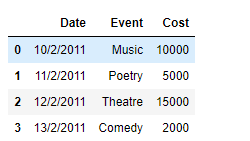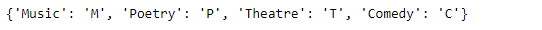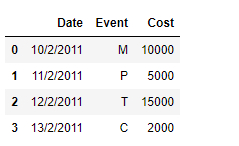# Using dictionary to remap values in Pandas DataFrame columns

• Last Updated : 23 Jan, 2019

While working with data in Pandas, we perform a vast array of operations on the data to get the data in the desired form. One of these operations could be that we want to remap the values of a specific column in the DataFrame. Let’s discuss several ways in which we can do that.

Given a Dataframe containing data about an event, remap the values of a specific column to a new value.

Code #1: We can use `DataFrame.replace()` function to achieve this task. Let’s see how we can do that.

 `# importing pandas as pd``import` `pandas as pd`` ` `# Creating the DataFrame``df ``=` `pd.DataFrame({``'Date'``:[``'10/2/2011'``, ``'11/2/2011'``, ``'12/2/2011'``, ``'13/2/2011'``],``                    ``'Event'``:[``'Music'``, ``'Poetry'``, ``'Theatre'``, ``'Comedy'``],``                    ``'Cost'``:[``10000``, ``5000``, ``15000``, ``2000``]})`` ` `# Print the dataframe``print``(df)`

Output :Now we will remap the values of the ‘Event’ column by their respective codes.

 `# Create a dictionary using which we``# will remap the values``dict` `=` `{``'Music'` `: ``'M'``, ``'Poetry'` `: ``'P'``, ``'Theatre'` `: ``'T'``, ``'Comedy'` `: ``'C'``}`` ` `# Print the dictionary``print``(``dict``)`` ` `# Remap the values of the dataframe``df.replace({``"Event"``: ``dict``})`

Output :Code #2: We can use `map()` function to achieve this task.

 `# importing pandas as pd``import` `pandas as pd`` ` `# Creating the DataFrame``df ``=` `pd.DataFrame({``'Date'``:[``'10/2/2011'``, ``'11/2/2011'``, ``'12/2/2011'``, ``'13/2/2011'``],``                    ``'Event'``:[``'Music'``, ``'Poetry'``, ``'Theatre'``, ``'Comedy'``],``                    ``'Cost'``:[``10000``, ``5000``, ``15000``, ``2000``]})`` ` `# Print the dataframe``print``(df)`

Output :Now we will remap the values of the ‘Event’ column by their respective codes.

 `# Create a dictionary using which we``# will remap the values``dict` `=` `{``'Music'` `: ``'M'``, ``'Poetry'` `: ``'P'``, ``'Theatre'` `: ``'T'``, ``'Comedy'` `: ``'C'``}`` ` `# Print the dictionary``print``(``dict``)`` ` `# Remap the values of the dataframe``df[``'Event'``]``=` `df[``'Event'``].``map``(``dict``)`` ` `# Print the DataFrame after modification``print``(df)`

Output :My Personal Notes arrow_drop_up